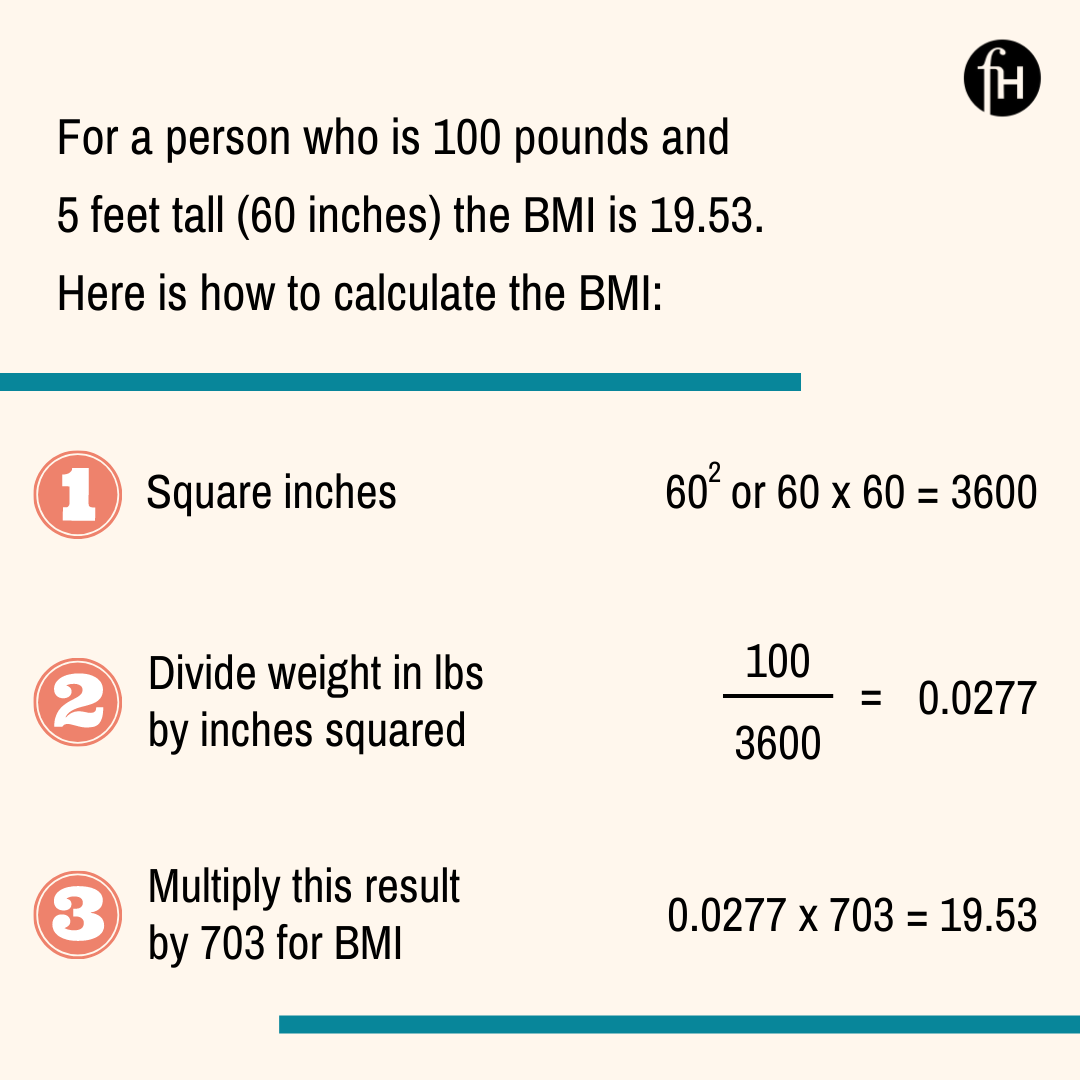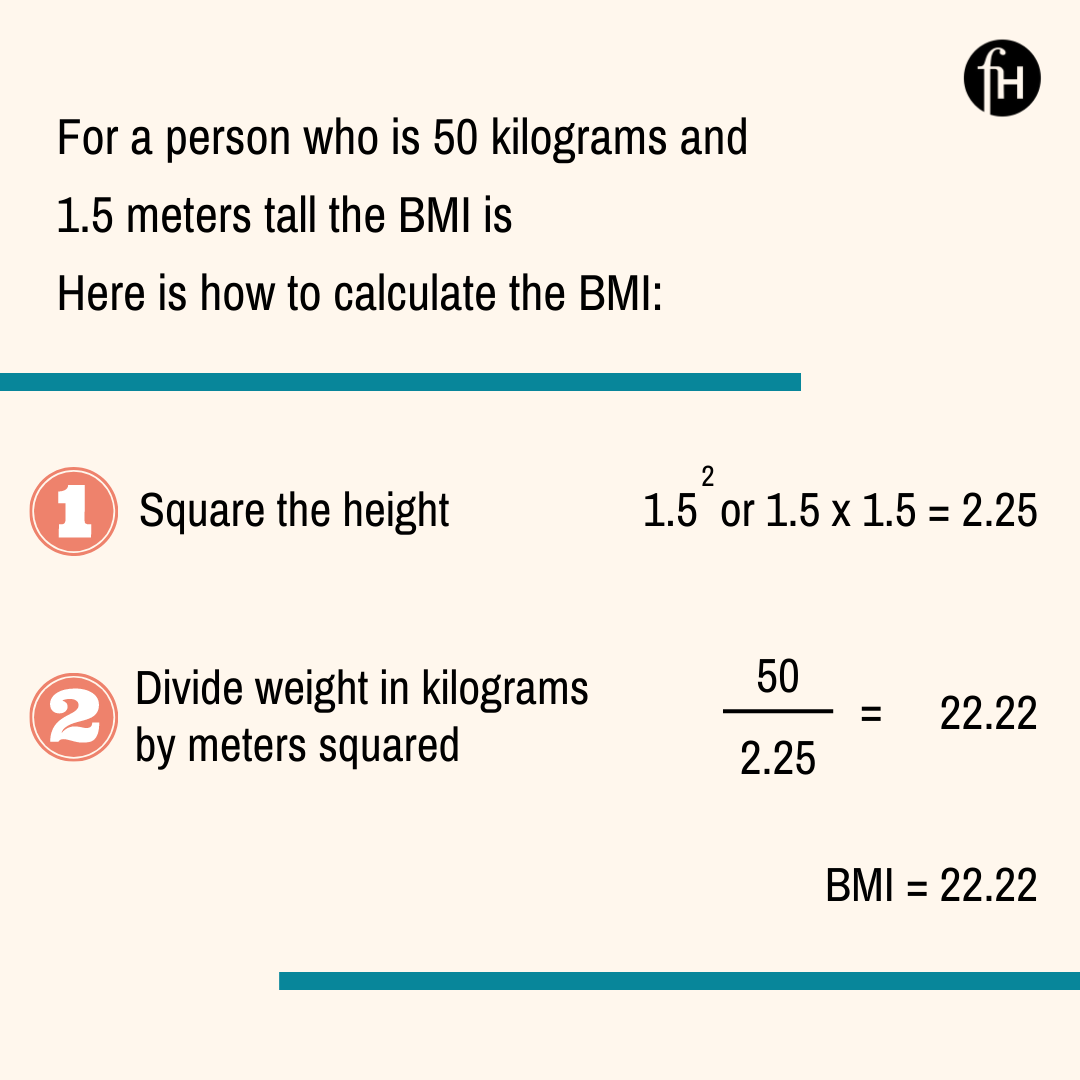# How Is Body Mass Index Calculated In Women

How BMI is calculated depends on whether the person's weight is in pounds or kilograms.

## How BMI Is Calculated For Weight In Pounds

Before calculating the BMI you will need to know a person's weight in pounds and height in inches. If the weight is in pounds, the height must be converted to inches. If the weight is in kilograms, the person's height must be converted to meters in order to use the formula.

You can calculate BMI by dividing weight (in pounds) by height (in inches) squared. Then, multiply this result by a conversion factor of 703. For example, for a person who weighs 100 lbs and has a height of 5 feet, you will first want to convert the height to inches. Five feet will be 60 inches. First, the number in pounds, which is 100, will be divided by 60 squared (or 60 x 60 which is 3600). The result of 100 divided by 3600 is 0.02777. This number is then multiplied by 703, for a resulting Body Mass Index of 19.53.

The equation for how BMI is calculated for weight in pounds and height in inches will look like below:## How BMI Is Calculated In Kilograms

The calculation for BMI in Kilograms is slightly different. You will have to convert the person's height and weight into the metric system. Then divide the weight in kilograms by the person's height in meters squared. So for someone who is 60 kilograms and 1.5 meters tall (which is about 5 feet tall), the calculation is as below:

### Related:Neon Yellow Pee - What Causes It?For the BMI calculation in kilograms there is no need to have the conversion factor of 703 that is required for calculating the BMI in pounds.

## How BMI Is Calculated: Take Home Points

Body Mass Index, abbreviated as BMI, is calculated the same way for all genders in people who are above 20 years old. If you are younger than 20 years old the calculation is different for men and women. There are two different formulas for calculating BMI. One formula requires that the weight be in pounds and the height be in inches. This formula also requires the use of a conversion factor of 703. The second formula is the formula to use if you have a person's weight in kilograms and their height in meters. If you do not want to do the calculation yourself, there are also calculators available online to do the conversion for you. BMI estimates do not apply to people who are pregnant. Finally, Body Mass Index is just a number. It needs to be used in context with a complete health assessment by your doctor.

Sources: